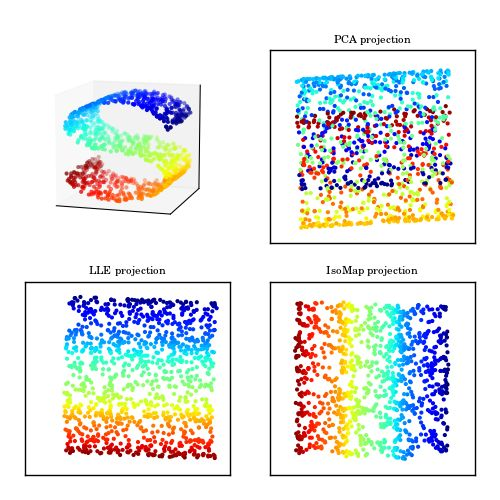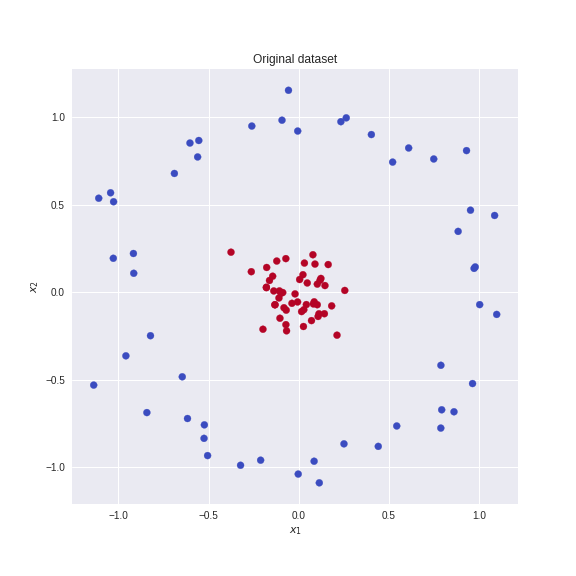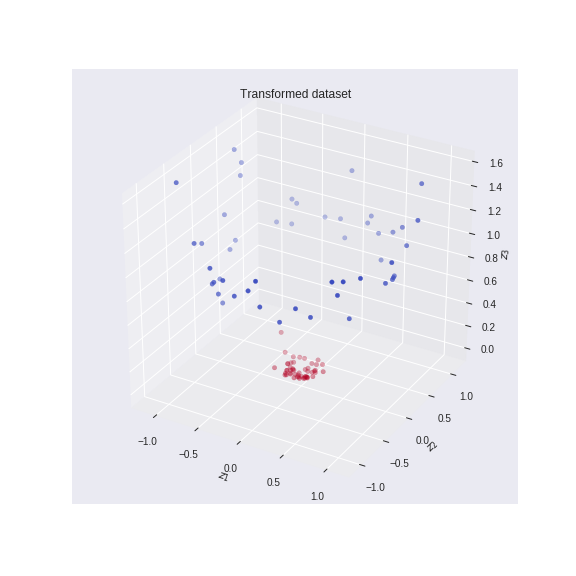×

Search anything:

# Kernel Principal Component Analysis (KPCA)

#### Machine Learning (ML) principal component analysis kernel principal component analysisGet this book -> Problems on Array: For Interviews and Competitive Programming

Reading time: 15 minutes

Kernel Principal Component Analysis (KPCA) is a non-linear dimensionality reduction technique. It is an extension of Principal Component Analysis (PCA) - which is a linear dimensionality reduction technique - using kernel methods.

# Why non-linear dimensionality reduction?

Sometimes the structure of the data is nonlinear, and the principle components will not give us the optimal dimensionality reduction, so we use non-linear methods like KPCA. For example in the figure below, PCA will look for the surface that will cross the S shaped data with minimal reconstruction error, but no matter what surface we choose, there will be some loss in information (Some points from different colors are mapped to one point. Unlike PCA, non-linear methods will give us more optimal projections (last two figures).# Intiution behind KPCA

The idea of KPCA relies on the intuition that many datasets, which are not linearly separable in their space, can be made linearly separable by projecting them into a higher dimensional space. The added dimensions are just simple arithmetic operations performed on the original data dimensions.

So we project our dataset into a higher dimensional feature space, and because they become linearly separable, then we can apply PCA on this new dataset.

Preforming this linear dimensionality reduction in that space will be equivalent to a non-linear dimensionality reduction in the original space.

For example consider this dataset where the brown and blue points are not linearly separable in two dimensions.We define the linear mapping T(x_1,x_2 )=(z_1,z_2,z_3 )=(x_1,x_2,x_1^2+x_2^2)
This mapping function will project the data from a lower dimensional (2D) to a higher dimensional (3D) space as shown in the figure below.We can see from the above figure that the dataset becomes linearly separable. The optimal surface that PCA will find will be in the middle between blue and brown points. And if we inverse this transform then this surface will correspond to a nonlinear curve in the original 2D space. And by projecting on that curve, we will find the optimal dimensionality reduction.

But doing PCA in high dimensional space will need a lot of computations, so in order to solve this problem we use kernel methods.

# Kernel Methods:

As we said doing PCA in the transformed dataset will need a lot of computations. But using kernel methods we can perform this computations in the original state space. This is done by kernel function which gives us a way of computing dot product, between two vectors - in high dimensional space- in our original space.

Some example of kernel functions are polynomial, Radial Basis Function (RBF) and Gaussian kernels.

# Steps of KPCA:

1. First we will choose a kernel functions k(x_i, x_j) and let T be any transformation to a higher dimension.

2. And like PCA, we will find the covariance matrix of our data. But here, we will use kernel function to calculate this matrix. So will compute kernel matrix, which is the matrix that results from applying kernel function to all pairs of data.
`K=T(X)T(X)^T`

``````                   k(x_1, x_1)  k(x_1, x_2) ........ k(x_1, x_d)
k(x_2, x_1)  k(x2, x_2)  ........ k(x_2, x_d)
K =           .           .                    .
.           .                    .
k(x_d, x_1)  k(x_d, x_2) ........ k(x_d, x_d)
``````

Note: KPCA scales with the size of our data.

1. Center our kerenl matrix (this equivalent to substract the mean of the transformed data and dividing by standard deviations) :
`K_new = K - 2(I)K + (I)K(I)`
where I is a matrix that its all elements are equal to `i/d`.
2. Then, we will find eigenvectors and eigenvalues of this matrix.
3. Sort our eigenvectors based on their corrsponding eigenvalues in a decreasing order.
4. We will choose what number of dimensions that we want our reduced dataset to be, let's call it m. Then we will choose our first m eigenvectors and concatenate them in one matrix.
5. Finally, Calculate the product of that matrix with your data. The result will be your new reduced dataset.

# Conclusion

In this post, you learned about KPCA and non-linear dimensionality reduction techniques in general. In practice, you should study your dataset very well, in order to select what algorithm you should use to reduce your dimensions. Also notice that KPCA takes more time than PCA.